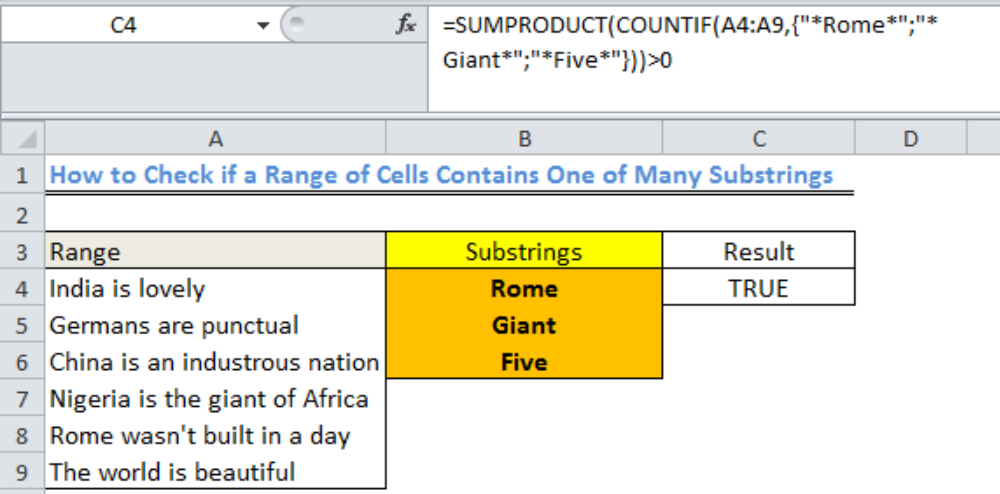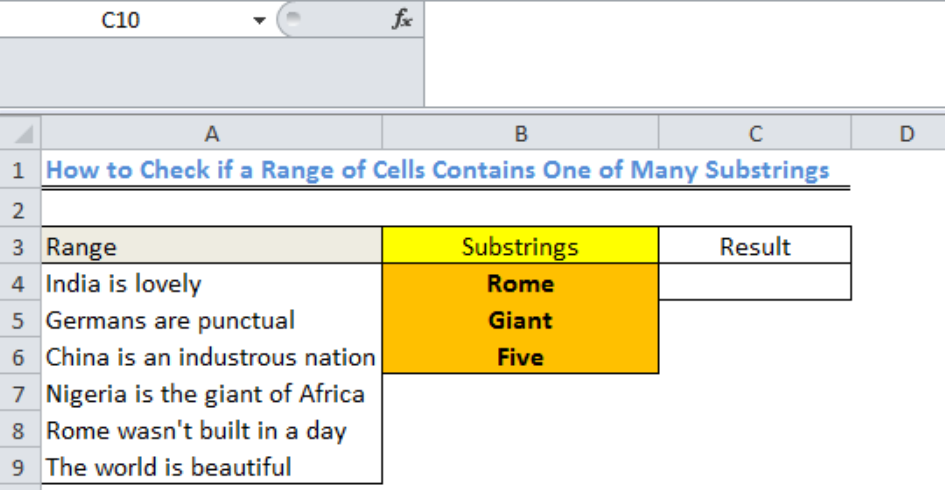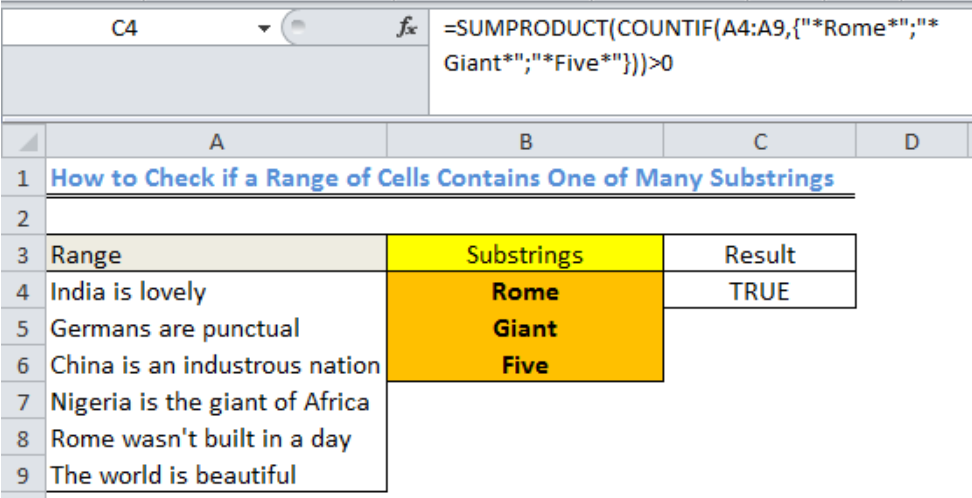Get instant live expert help with Excel or Google Sheets“My Excelchat expert helped me in less than 20 minutes, saving me what would have been 5 hours of work!”

#### Post your problem and you’ll get expert help in seconds.

Your message must be at least 40 characters
Our professional experts are available now. Your privacy is guaranteed.

# How to Check if a Range Contains One of Many Substrings

We can check if a range of cells contains one of many substrings with the SUMPRODUCT and COUNTIF functions. This article will walk through the process.Figure 1: How to Check if a Range of Cells Contains One of Many Substrings

## Formula

`=SUMPRODUCT(COUNTIF(A4:A9,{"*Rome*";"*Giant*";"*Five*"}))>0`

## Setting up the Data

• We will input the data into the table as shown in figure 2
• We will check the range (A4:A9) if they contain any of the substrings in B4:B6Figure 2: Data to Check if a Range of Cells Contains One of Many Substrings

## Check if a Range of Cells Contains One of Many Substrings

• We will click on Cell C4
• We will input the formula below into the cell

`=SUMPRODUCT(COUNTIF(A4:A9,{"*Rome*";"*Giant*";"*Five*"}))>0`

• We will press the enter key to get the resultFigure 3: Result for the Range of Cells Showing that it Contains Rome and Giant

## Explanation

The COUNTIF function counts the contents of the substrings that appear within the chosen RANGE (A4:A9). Because the COUNTIF function is laden with multiple values to search for, every count for a value is received in the form of 0 and 1.

The SUMPRODUCT function returns as TRUE any value that is greater than ZERO.

## Instant Connection to an Expert through our Excelchat Service

Most of the time, the problem you will need to solve will be more complex than a simple application of a formula or function. If you want to save hours of research and frustration, try our live Excelchat service! Our Excel Experts are available 24/7 to answer any Excel question you may have. We guarantee a connection within 30 seconds and a customized solution within 20 minutes.

### Did this post not answer your question? Get a solution from connecting with the expert.Another blog reader asked this question today on Excelchat:
Related blogs
Solution examplesi have a table with Dates, First names , Last names and i want to count how many times a name occurs, but if a name occurs more than once on a particular date i need to only count it once. this is on excel and not google sheets
Solved by F. H. in 31 minsI need a formula to count the number of rows that contain at least one of two possible phrases, but not give me the total number of instances that both phrases occur overall.
Solved by E. B. in 33 minsI want to be able to alter the appearance (font color and fill) of a column of numbers using conditional formatting, based on being greater than or less than the numbers from another column. I am using Excel 2016. I am unable to get any formula to work. Please help!
Solved by I. J. in 59 minsI need assistance with an excel formula. I have a 12 month sheet that has a summary page on the 13 page. the monthly totals changes so on the summary page I want to sum the difference - eg. if Jan total is 1500, feb total is 1000, mar total is 800, I want the summary page to show the current amount of 800 when you place the formula to capture all months---showing the diminishing value rather than the sum of all the pages
Solved by E. L. in 60 minshow can i turn my sumifs into a countifs because my sumifs works great and i also want to count the thing im summing
Solved by O. C. in 40 mins## Subscribe to Excelchat.coAnother blog reader asked this question today on Excelchat: